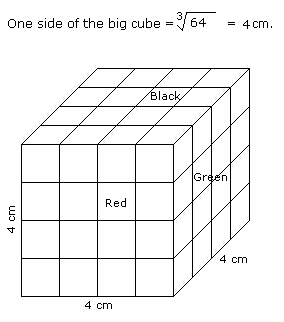# Verbal Reasoning - Cube and Cuboid - Discussion

Discussion Forum : Cube and Cuboid - Cube and Cuboid 5 (Q.No. 4)
Directions to Solve

The following questions are based on the information given below:

All the opposite faces of a big cube are coloured with red, black and green colours. After that is cut into 64 small equal cubes.

4.
How many small cubes are there whose only one face is coloured ?
32
8
16
24
Explanation:Number of small cubes having only one face coloured = 4 from each face

= 4 x 6

= 24

Discussion:
2 comments Page 1 of 1.

Pranay said:   9 years ago
Other way of solving.

We have 3 formula for for same problem like one or 2 or 3 faces:

No side colored = (n-2)^3.

One side colored = 6*(n-2)^2.

2 side colored = 12*(n-2).

3 sided colored for cube always 8.

Sribash biswas said:   1 decade ago
If all the side is colored thenn the formula is

For only one side color = 6* (segment line -2) ^2.